Courses

# Design Of Welded Joints Mechanical Engineering Notes | EduRev

## Mechanical Engineering : Design Of Welded Joints Mechanical Engineering Notes | EduRev

The document Design Of Welded Joints Mechanical Engineering Notes | EduRev is a part of the Mechanical Engineering Course Machine Design.
All you need of Mechanical Engineering at this link: Mechanical Engineering

Design of a butt joint:

The main failure mechanism of welded butt joint is tensile failure. Therefore the strength of a butt joint is

P = sT lt

where sT =allowable tensile strength of the weld material.

t =thickness of the weld

l =length of the weld.

For a square butt joint is equal to the thickness of the plates. In general, this need not be so (see figure 1).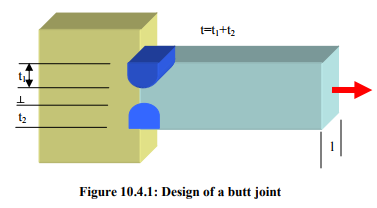Design of transverse fillet joint:

Consider a single transverse joint as shown in figure 10.4.2. The general stress distribution in the weld metal is very complicated. In design, a simple procedure is used assuming that entire load P acts as shear force on the throat area, which is the smallest area of the cross section in a fillet weld. If the fillet weld has equal base and height, (h, say), then the cross section of the throat is easily seen to be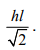With the above consideration the permissible load carried by a transverse fillet weld is

P = ss Athroat

where ss -allowable shear stress

Athroat  =throat area.

For a double transverse fillet joint the allowable load is twice that of the single fillet joint.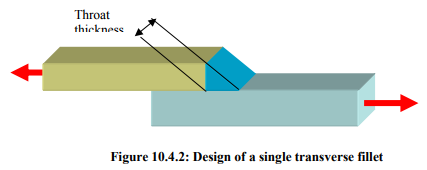Design of parallel fillet joint:

Consider a parallel fillet weld as shown in figure 10.4.3. Each weld carries a load P/2 . It is easy to see from the strength of material approach that the maximum shear occurs along the throat area (try to prove it). The allowable load carried by each of the joint is st Awhere the throat area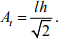The total allowable load is

P = 2 ss At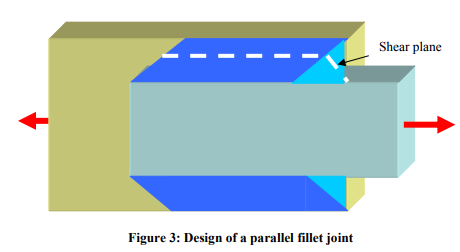In designing a weld joint the design variables are and . They can be selected based on the above design criteria. When a combination of transverse and parallel fillet joint is required (see figure-10.4.4) the allowable load is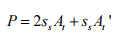where At =throat area along the longitudinal direction.

At ' =throat area along the transverse direction.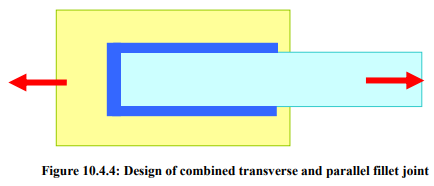Design of circular fillet weld subjected to torsion:

Consider a circular shaft connected to a plate by means of a fillet joint as shown in figure-10.4.5. If the shaft is subjected to a torque, shear stress develops in the weld in a similar way as in parallel fillet joint. Assuming that the weld thickness is very small compared to the diameter of the shaft, themaximum shear stress occurs in the throat area. Thus, for a given torque the maximum shear stress in the weld is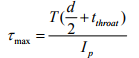where T =torque applied.

d =outer diameter of the shaft

t throat = throat thickness

Ip =polar moment of area of the throat section.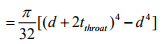where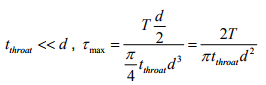The throat dimension and hence weld dimension can be selected from the equation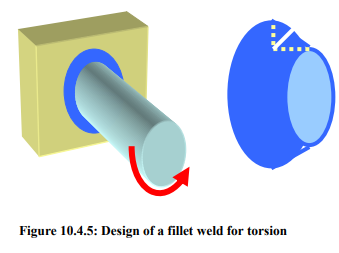Design stresses of welds:

Determination of stresses in a welded joint is difficult because of

inhomogeneity of the weld joint metals
thermal stresses in the welds
changes of physical properties due to high rate of cooling etc.

The stresses in welded joints for joining ferrous material with MS electrode are tabulated below.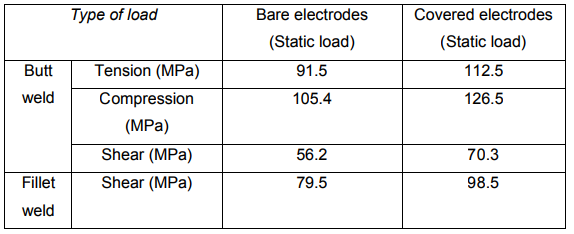Offer running on EduRev: Apply code STAYHOME200 to get INR 200 off on our premium plan EduRev Infinity!

## Machine Design

56 videos|71 docs|94 tests

,

,

,

,

,

,

,

,

,

,

,

,

,

,

,

,

,

,

,

,

,

;